`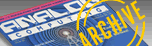A.N.A.L.O.G. ISSUE 62 / JULY 1988 / PAGE 12`

 ANALOG Man is the editor of the famous ANALOG Computing Magazine, the premier magazine for Atari users. His job is to assemble the pages of each issue, which he does by running over the pages, causing them to fall to the level below in the girder-like offices of ANALOG Mag. You must help Man do his job of assembling nine issues of ANALOG by guiding his footsteps with your joystick plugged into port 1. He can climb up and down ladders, and falling down the holes left by runover pages doesn't hurt a bit. . . Man is tough. 48k disk or cassette Analog Man by Dvid Plotkin     Of course, there is far more to it than just happily showing up at the office every day. The other personal computers are getting more and more nervous with the success of ANALOG and Atari, and they have decided the way to finish Atari for good is to prevent ANALOG from reaching its loyal readers. So one day, they showed up at ANALOG's offices and began chasing poor Man. Their touch deprives Man of one of his five lives. But Man is not defenseless. To combat the evils of the enemy personal computers, Man carries five bombs. Pressing the button on your joystick sets off a bomb, and any enemy who touches a bomb is instantly frozen and can do no further harm until he unfreezes. There are nine different levels to ANALOG Man, and everything gets faster after you complete the first nine screens. Getting through all nine screens earns you two additional bombs, up to a maximum of ten. Oh, yes-the enemies stay frozen a shorter length of time in the upper levels ... So get busy, loyal readers of ANALOG, and help ANALOG Man get the issues of your favorite magazine out on time. R u n n i n g ANALOG Man     ANALOG Man is too long to compile from memory. Punch it in exactly as listed (using D:CHECK IN ACTION! from issue 44 to check your typing), then save it to disk (using the SHIFT-CNTRL-W command). Go to the monitor (SHIFT-CNTRL-M) and reboot the system to clear memory (B). Reenter the monitor and type: C "D:FILENAME. " When the compile is done, simply type R to run the program. Program Take-apart     Some of the more interesting procedures are listed below, with a word of explanation on how they work. Much can be learned from studying the structured Action! listing.     PROC DOWNLOAD: The screens for this game are constructed using a redefined character set in Antic mode 4, the multicolored character mode. This procedure steps back the top of memory and moves the character set from ROM into RAM so it can be modified.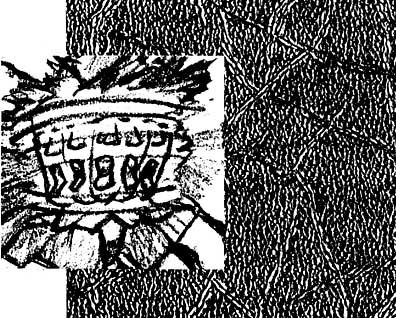PROC DLINT: ANALOG Man uses a display list interrupt (DLI) to get extra color on the screen. The numbers with dollar signs in front of them are hex codes for the machine language equivalent of the commands to put the contents of the accumulator, X and Y registers on the stack and pop them back off. The balance of this procedure is simply to wait for the horizontal synch, then change the contents of the text window color register and the intensity of the text in the window.     PROC SCORELINE: Setting up the DLI defined in DLINT places the address of DLINT into the card variable Vdslst, which resides at locations 512 and 513. Whenever a DLI is required, the Atari checks the contents of these locations to find the address of the routine to execute for the DLI. It will now use Dlint. Byte array Dlist was "pointed" to the same place in memory as the display list, so changing one of the elements of Dlist will change the display list, thus calling the DLI at the required line. The DLI is actually turned on by placing hex \$C0 into location NMIEN (\$D40E).     PROC MOVEIT: Byte array Adres is pointed to the address defined by the PmAdr function, offset by the y coordinate of the Player in question. Then num bytes of array Shape are moved to this address using the built-in MOVEB-LOCK command. Finally, the x coordinate of the Player is set by changing one of the elements of byte array PmHpos, which has been defined to reside at the memory locations that the Atari uses to set the horizontal locations of the Players (\$D000).     PROC TESTCOL: This procedure tests for collisions between Players, for use in PROC PMHIT. Testing for collisions in a language as fast as Action! can be a little tricky. Whenever it becomes necessary to look for a collision between two Players, you must wait for the entire screen to be drawn, so that collisions will be registered. This is the purpose of waiting for VCount AND 128. The problem is that if you need to check for collisions several times in the course of one program loop, as you do in ANALOG Man, the waiting for the complete screen to be drawn before checking for the collision will considerably slow down the game. The solution is to check the hardware registers for collisions only once in each loop, store the results of the check in temporary holding registers, and use the temporary registers for all further work. TESTCOL uses this technique. Of course, you must clear the temporary registers before each collision check, and clear the hardware registers (PmHitClr = 1) after each check.     PROC TITLE: The rolling colors of the title screen are created by storing colors directly into the hardware color registers. The color to store is based on the timer located at memory register 20, which "ticks" every 1/60 of a second. Since 60 times per second is too fast to change the color (it doesn't look very nice), the number in the timer is divided by 4 (RSH 2). The result is then added to the scan line counter, VCount, so that each scan line is a different color, and the rolling rainbow effect is based on the timer. By subtracting one of the two numbers generated by the above method from 128, the colors of that register appear to roll backward. By avoiding the use of the DLI, you can have multiple colors within each letter-something most people will tell you can't be done on the Atari.     PROC GR4INIT: This procedure sets up the necessary information for use in the custom PLOT and LOCATE routines to come later. The elements of card array Linept are equated to the address of the beginning of each screen line. Then byte array Dlist is pointed to the Display list by equating Dlist to Sdlst, which is a card variable residing at locations 560 and 561, the registers which contain the address of the display list. Finally, the display list is modified to Antic mode 4 by changing the elements of Dlist.     PROC PLOT4: This is a custom PLOT routine, far faster than the one built into the Action! cartridge. Byte array Line is equated to an element of card array Linept. Then an element of Line is modified to place the required character on the screen. LOCATE4 works similarly, except the element of Line is simply returned instead of being modified.     PROC SQUASHED: This procedure checks to see if a falling level has hit one of the enemies. Note the conversion from Playfield coordinates to Player coordinates in order to do this check.     PROC NOCHASE: This procedure and PROC CHASE control the movement of the enemy Players. If the distance between ANALOG Man and his enemies is too great, they will not "see" him, and will move randomly. However, if they get close, they will begin to follow him, and the only escape may be to use a bomb. The distance at which the enemies will begin to follow Man gets greater as you get to higher levels.     PROC VECTOR: This procedure doesn't seem to do anything, since it contains nothing but a RETURN. In fact, it is very important in determining what level will appear on the screen. The problem that I faced was that if you get killed in the middle of a game (highly likely!), it is very unwieldy to get back to level 1 if you decide to play again.     In fact, the whole coding scheme was unwieldy, looking something like this: Screenl (), Play (), Screen2 (), Play (), etc ... Instead, the address of each procedure to draw a screen (Screen1, etc.) is stored into the elements of card array SC in the last procedure of the program, Main. Then, Vector is simply equated to the appropriate element of SC, so now Vector points to the procedure to draw a screen instead of to the dummy procedure that does nothing. Calling Vector now executes the procedure to draw a screen. S u m m a r y     ANALOG Man is a rather long program, but it would have been considerably longer and more confusing if the powerful capabilities to relocate arrays and even procedures had not been used. I think you can see that Action! is one of the most powerful languages ever developed for any home computer. I recommend that if you are serious about your Atari, you support the developers of Action! and purchase a copy of this outstanding language.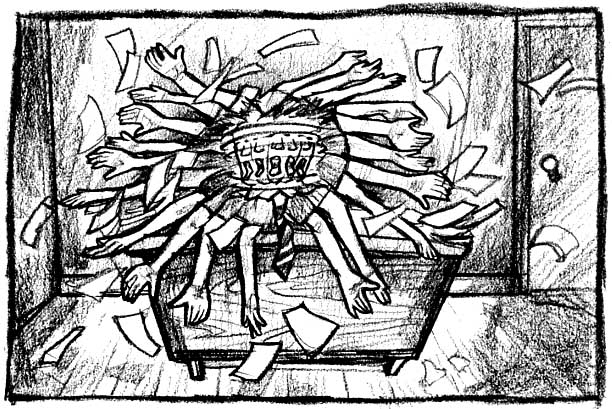Listing 1 : Action! ;ANALOG MAN by David Plotkin ; ;COPYRIGHT 1988 ;BY ANALOG COMPUTING ;     CHECKSUM DATA ;[56 57 F8 33 3E 56 EC CC ; B7 CD B7 6D FA 21 D4 D9 ; 2F D7 9A 8B CB 11 75 44 ; D9 E9 43 C6 BB EB D0 11 ; CB 34 7F 69 98 7D C4 05 ; 0D 2A 95 C7 8A 67 19 F1 ; 1C 79 6F 6C BF C8 9D 92 ; 44 BC CA 83 95 B9 61 0B ; 0C 40 E8 FA 15 63 C1 43 ; 57 BE 36 37 69 D0 9F D6 ; BD 22 31 70 06 33 3C 2A ; E4 2D 8D 6C 09 C9 73 ] MODULE BYTE ChrBase=756,Bkgrnd=710,X,Y,      Fate=53770,Level=,CursIn=752,      Stick0=632,Ps,Loud=,Indx=[O],      Snd1=\$D208,Snd2=\$D20F,Freq=,      Wsync=\$D40A,Colbk=\$D018,      NMien=\$D40E,COnsol=53279,      Colints=\$D017,X0,Y0,Ft=,      Lv=,Ld=,Ld2=[O],Atrt=77,      PMHitClr=\$D01E,Dmactl=\$22F,      Gractl=\$D01D,PMBase=\$D407,      Priority=\$26F,Vcount=54283,      Loud1=,Tone=,Flg=,      Mstatus=,Pep=,My= CARD Scrn=88,RamSet,HiMem=\$2E5,      Score=,Sdlst=560,      Vdsist=512,Max=,      Pm_BaseAdr,Adres,AdresB INT Kdir,Ydir INT ARRAY Pxdr=[0 0 0 0],           Pydr=[0 0 0 0] CARD ARRAY Linept(24),Sc(10) BYTE ARRAY Charset,Dlist,Stacky(9),      PmHpos(8)=\$D008,Stack(9),      Stackx(7)=[0 9 25 9 26 9 26],      Px(4)=[0 0 0 0],Py(4)=[0 0 0 0],      Begx(4)=[0 64 124 184],      Begy(4)=10 18 90 42],      Pm_Width(5)=\$D008,Plptr      Pm_Mismask(4)=[\$FC \$F3 \$CF \$3F],      Pcolr(4)=704,Pmtopf(8)=\$D000,      Pmtop(8)=\$D008,Pfcol(8),PCOl(8),   Chmp1(0)=[0 0 0 0 28 42 54 28 73 127   28 20 22 48 0 0 0 0],   Chmp2(0)=[0 0 0 0 28 42 54 28 8   127 93 20 52 6 0 0 0 0],   Ibm(0)=[0 0 0 0 88 84 88 84 88 0 68   108 84 68 0 0 0 0],   CMdore(0)=[0 0 0 0 224 176 151 134   128 128 134 151 176 224 0 0 0 0],   Apple(0)=[0 0 0 0 48 8 8 62 127 127   127 127 62 28 8 8 0 0],   Estat(4),   Msl1(0)=[170 85 170 85 170 85 170 85   170 85 170 85 170 85 170 85 170 85],   Msl2(0)=[85 170 85 170 85 170 85 170   85 170 85 170 85 170 85 170 85 170],   ShapeTabie(0)=[   85 17 17 68 68 17 17 85;1-GIRDER   160 170 160 160 160 160 170 160;   10 170 10 10 10 10 170 10;3 RT LDR   170 51 85 51 170 51 85 51;4 LV1   0 0 0 0 170 85 170 85;5 LV1 CRUNCH   85 51 170 51 85 51 170 51;6 LV2   0 0 0 0 85 170 85 170;7 LV2 CRUNCH   255 34 85 34 255 34 85 34;8 LV3   0 0 0 0 255 85 255 85;9 LV3 CRUNCH   165 51 90 51 165 51 90 51;10 LV4   0 0 0 0 165 90 165 90;11 LV4 CRUNCH     190 235 215 195 195 215 235 190;12     235 130 150 170 170 150 130 235;13     ] PROC Pause() ;TEST WHILE Consol<>6 DO OD RETURN PROC Download() ;Step back HiMeM and move the ;character set into RAM RaMSet=(HiMem-\$400)&\$FC00;1K boundary ChrBase=RamSet RSH 8 HiMem=RamSet MoveBlock(RamSet,57344,1024) Charset=RamSet RETURN PROC Modify() ;Modify the RAM character set CARD xx FOR xx=0 TO 103 DO   Charset(xx+8)=ShapeTable(xx) OD RETURN PROC Pmgraphics() Zero(PmHpos,8) Zero(Pm_Width,5) Dmactl=\$2E Pcolr(0)=52 Pm_BaseAdr=(HiMem-\$400)&\$FC00 PmBase=Pm_BaseAdr RSH 8 HiMem=Pm_BaseAdr+384 Priority==&\$C0%1 Gractl=3 RETURN CARD FUNC PmAdr(BYTE n) IF n>=4 THEN n=0 ELSE n==+1 FI RETURN(Pm_BaseAdr+384+(n*\$80)) PROC PmClear(BYTE n) CARD ctr BYTE ARRAY playadr playadr=PmAdr(n) IF n<4 THEN Zero(playadr,\$80)  ELSE n==-4 FOR ctr=0 TO \$80-1 DO playadr(ctr)==&Pm_Mismask(n) OD FI RETURN PROC Dlint() ;the display list interrupt routine [S48 \$8A \$48 \$98 \$48] Wsync=1 Colbk=50 Colints=12 [\$68 \$A8 \$68 \$AA \$68 \$40]   PROC ScoreLine() ;set up the dli Vdslst=Dlint Dlist(27)=132 Nmien=SC0 RETURN PROC Moveit(BYTE ARRAY shape BYTE             which,nuM,xx,yy) Adres=PmAdr(which)+yy MoveBlock(Adres,shape,num) PmHpos(which)=xx RETURN PROC Putman() ;Clear PM space/ put Players onscreen BYTE lp FOR lp=0 TO 3 DO   Estat(lp)=0 PmClear(lp) OD Mstatus=0 Ld=0 Ld2=0 SndRst() X0=76 Y0=66 Moveit(Chmp1,0,18,X0,Y0) FOR lp=1 TO 3 DO  Px(lp)=Begx(lp) Py(lp)=Begy(lp)  IF lp=1 THEN   Moveit(Ibm,1p,18,Px(1N),Py(lp))   ELSEIF lp=2 THEN   Moveit(CMdore,lp,18,Px(lp),Py(lp))   ELSE   Moveit(Apple,lp,18,Px(lp),Py(lp))  FI OD RETURN PROC Testcol() BYTE ll FOR ll=0 TO 7 DO Pfcol(ll)=0 Pcol(ll)=0 OD DO UNTIL Vcount&128 OD FOR ll=0 TO 7 DO Pfcol(ll)=Pmtopf(ll) Pcol(ll)=Pmtop(ll) OD PmHitClr=1 RETURN BYTE FUNC PmHit(BYTE n,(num) IF n<4 THEN n==+4 ELSE n==-4 FI IF (num(4 THEN  RETURN((Pcol(n) RSH (num)&1)  ELSE (num==&3  RETURN((Pfcol(n) RSH (num)&1) FI RETURN(0) PROC Msldrop () ;put Pepper on screen BYTE trig=644,lp,tt= IF Ld>1 THEN Ld==-2   Sound(2,Ld LSH 3,10,Ld) ELSEIF   Mstatus>0 THEN   Sound(2,Mstatus LSH 2,10,4) FI IF Mstatus>0 THEN tt=1-tt Mstatus==+1   IF tt=0 THEN     MoveBlock(AdresB,Ms12,18) ELSE     MoveBlock(AdresB,Msl1,18)   FI   IF Mstatus=50 THEN Zero(AdresB,18)     Mstatus=0 Sound(2,0,0,0)   FI FI IF trig=1 OR Pep=0 OR Mstatus>0  THEN RETURN FI Mstatus=1 FOR lp=0 TO 3 DO PmHpos(lp+4)=X0-3+(lp LSH 2) OD My=Y0 AdresB=PmAdr(4)+My MoveBlock(AdresB,Ms11,18) Ld=12 Pep==-1 Position(36,23) Print("   ") Position(36,23) PrintB(Pep) RETURN PROC Gotbumped() BYTE lq,lg1 IF Ld2>0 THEN Ld2==-1 FI Sound(3,Ld2 LSH 3,8,Ld2) FOR lq=0 TO 3 DO FOR lq1=1 TO 3 DO IF PmHit(lq+4,lg1)=1 AND Estat(lg1)=0  THEN Ld2=14 Estat(lg1)=l Score==+5  PmHpos(lq+4)=0 FI OD OD FOR lq=1 TO 3 DO IF Estat(lq)>0 THEN Estat(lq)==+1  Pcolr(lq)=((Rand(14)+1) LSH 4)+10 FI IF Estat(lq)=Ft THEN Estat(lq)=0  PmClear(lq)  Pcolr(lq)=((Rand(14)+1) LSH 4)+10  Px(lq)=Begx(lq) Py(lq)=Begy(lq)  IF lq=1 THEN   Moveit(Ibn,lq,18,Px(lq),Py(lq))   ELSEIF lq=2 THEN   Moveit(Cmdore,lq,18,Px(lq),Py(lq))   ELSE   Moveit(Apple,lq,iB,Px(lq),Py(lq))  FI FI OD RETURN PROC Title() BYTE colpf0=53270,colpf1=53271,      colpf3=53273,rtclock=20 Graphics(18) Position(5,4) PrintD(6,"ANALOG MAN") Position(8,5) PrintD(6,"BY") Position(3,7) PrintD(6,"david Plotkin") Position(3,9 PrintDt6,"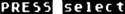") WHILE Consol<>5 DO colpf3=Fate Atrt=0 Wsync=0   colpf0=128-Vcount+rtclock RSH 2   colpf1=Vcount+rtclock RSH 2 OD RETURN PROC Gr4Init() ;Set up the address of each screen ;line,initialize and set up Gr. 4 CARD xx BYTE clr1=709 Graphics(0) CursIn=1 Print(" ") FOR xx=0 TO 23 DO Linept(xx)=Scrn+(40*xx) OD Dlist=Sdlst Dlist(3)=68 FOR xx=6 TO 27 DO D1ist(xx)=4 OD clr1=68 RETURN PROC Update() ;print data on the text line Position(0,23) Print("Score: ") Position(7,23) PrintC(Score) Position(13,23) Print("Lives: ") Position(20,23) PrintB(Lv) Position(22,23) Print("Hi: ") Position(26,23) PrinTC(Max) Position(32,23] Print("SB: ") Position(36,23) Print("   ") Position(36,23) PrintB(Pep) RETURN PROC P1ot4(BYTE x,y,ch) ;Plot a char at location x,y BYTE ARRAY line line=Linept(y) line(x)=ch RETURN BYTE FUNC Locate4(BYTE x,y) ;Returns the value of the char at x,y BYTE ARRAY line line=Linept(y) RETURN (line(x) PROC Hline(BYTE x1,y1,x2,ch) ;draw a line of ch characters from ;x1,y1 to x2,y1 (horizontal line) BYTE ARRAY line BYTE lp line=Linept(y1) lp=x1 DO line(lp)=ch lp==+1 UNTIL lp=x2+1 OD RETURN INT FUN( HStick(BYTE port) BYTE ARRAY ports(4)=\$278 INT ARRAY value(4)=(0 1 \$FFFF 0] port==&3 RETURN (value((ports(port)&\$C) RSH 2)) INT FUNC VStick(BYTE port) BYTE ARRAY portsC4)=\$278 INT ARRAY value(4)=[0 1 \$FFFF 0] port==&3 RETURN (value(ports(port)&3)) PROC EndGame () ;game over BYTE trig=644,wsync=\$D40A,rtclock=20,      lm=53271,vcount=54283 SndRst() Bkgrnd=0 Dlist(10)=2 IF Score>Max THEN Max=Score FI Put (125) Update() Position(7,5) Print("All DONE Press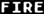") DO vcount=0 lm=vcount+rtclock RSH 2   Atrt=0 UNTIL trig=0 OD Bkgrnd=148 Dlist(10)=4 Put(125) Lv=5 Pep=5 Indx=0 Level=5 Ft=200 Score=0 Update() PmHitClr=0 RETURN PROC Meltdown() BYTE lp,lq,time=20 BYTE ARRAY melt SndRst() melt=PmAdr(0)+Y0+4 FOR lp=0 TO 30 DO lq=Rand(10) melt(lq)=Fate  Sound(0,Fate,8,8)  time=0 DO UNTIL time=3 OD OD FOR lp=0 TO 9 DO melt(lp)=8 Sound(0,lp*10,10,8)  time=0 DO UNTIL time=2 OD OD Sound(0,0,0,0) RETURN PROC Ouch() BYTE lc,ld IF Pcol(4)=0 THEN RETURN FI FOR lc=1 TO 3 DO IF PmHit(0,lC)=1 AND Estat(lc)>0  THEN RETURN FI OD Meltdown() FOR lc=O TO 7 DO PmClear(lc) OD Lv==-1 Position(20,23) PrintB(Lv) IF Lv=0 THEN EndGame() ELSE Putman() PmHitClr=0 FI RETURN PROC InitLev() ;Set initial stack values, call Putman BYTE lp FOR lp=1 TO 8 DO Stack(lpl=0 OD Stacky(1)=4 Stacky(2)=4 Stacky(3)=10 Stacky(4)=10 Stacky(5)=16 Stacky(6)=16 Stacky(7)=0 Stacky(8)=0 PutMan() RETURN PROC Girders() ;draw the main four lines of girders ;clear screen and init new level SndRst() Zero(Scrn,960) Loud=0 Hline(2,22,37,1) Hline(2,16,37,1) Hline(2,10,37,1) Hline(2,4,37,1) Hline(9,4,13,4)  H1ine(9,10,13,6) Hline(9,16,13,8) Hline(26,4,30,4) Hline(26,10,30,6) Hline(26,16,30,8) InitLev() RETURN PROC Screen1() ;draw screen 1 BYTE lp Girders();now the ladders FOR lp=4 TO 21 DO Plot4(2,lp,2) Plot4(3,lp,3)   Plot4(19,lp,2) Plot4(20,lp,3)   Plot4(36,lp,2) Plot4(37,lp,3) OD Position(15 23) Print ("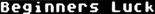") RETURN PROC 5creen2() ;draw screen 2 BYTE lp Girders() FOR lp=4 TO 21 DO Plot4(19,lp,2) Plot4(20,lp,3) OD FOR lp=18 TO 15 DO Plot4(2,lp,2) Plot4(3,lp,3) OD Position(15,23) Print ("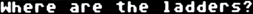") RETURN PROC Screen3() ;draw screen 3 BYTE lp Girders() FOR lp=4 TO 21 DO Plot4(19,lp,2) Plot4(20,lp,3) OD FOR lp=4 TO 9 DO Plot4(2,lp,2) Plot4(3,lp,3) OD FOR lp=16 TO 21 DO Plot4(36,lp,2) Plot4(37,lp,3) OD Position(15 23) Print ("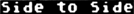") RETURN PROC Screen4() ;draw screen 4 BYTE lp Girders() Hline(16,4,23,0) Hline(16,16,23,0) FOR lp=4 TO 21 DO Plot4(14,lp,2) Plot4(15,lp,3)   Plot4(24,lp,2) Plot4(25,lp,3) OD Position(15 23) Print ("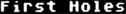") RETURN PROC Screen5() ;draw screen 5 BYTE lp Girders() Hline(16,10,23,0) Hline(16,16,23,0) FOR lp=4 TO 21 DO P1ot4(19,lp,2) Plot4(20,lp,3) OD FOR lp=4 TO 9 DO Plot4(14,lp,2) Plot4(15,lp,3)   P1ot4(24,lp,2) Plot4(25,lp,3) OD FOR lp=16 TO 21 DO Plot4(14,lp,2) Plot4(15,lp,3)   Plot4(24,lp,2) Plot4(25,lp,3) OD Position(15,23) Print("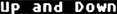") RETURN PROC Screen6() ;draw screen 6 BYTE lp Girders() Hline(16,4,23,8) Hline(16,10,23,0) Hline(16,16,23,0) FOR lp=4 TO 21 DO Plot4(14,lp,2) Plot4(15,lp,3)   Plot4(24,lp,2) Plot4(25,lp,3) OD Position(15,23) Print ("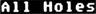") RETURN PROC Screen)() ;draw screen 7 BYTE lp Girders() Hline(16,10,23,0) Hline(16,16,23,0) FOR lp=4 TO 21 DO Plot4(19,lp,2) Plot4(28,lp,3) OD Position(15,23) Print ("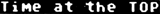") RETURN PROC Screen8() ;draw screen 8 BYTE lp Girders() Hline(16,19,23,0) Hline(16,16,23,0) FOR lp=4 TO 21 DO Plot4(2,lp,2) Plot4(3,lp,3)   Plot4(36,lp,2) P1ot4(37,lp,3) OD Position(15,23) Print ("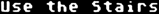") RETURN PROC Screen9() ;draw screen 9 BYTE lp Girders() Hline(16,4,23,0) Hline(16,10,23,0) Hline(16,16,23,0) FOR lp=4 TO 21 DO Plot4(2,lp,2) Plot4(3,lp,3)   Plot4(36,lp,2) Plot4(37,lp,3) OD Position(15,23] Print ("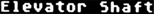") RETURN PROC Falling(BYTE tt) ;keep track of level status BYTE lp IF tt=4 THEN   IF X0<120 THEN Stack(1)==+1 ELSE     Stack(2)==+1 RETURN   FI FI IF tt=6 THEN   IF X0<120 THEN Stack(3) ==+1 ELSE     Stack(4)==+1 RETURN   FI FI IF tt=8 THEN   IF X0<120 THEN Stack(5)==+1 ELSE     Stack(6)==+1 RETURN   FI FI RETURN PROC Squashed(BYTE wh) BYTE lk,xx,yy xx=(Stackx(wh) LSH 2)+48 yy=CStacky(wh) LSH 2)+16-14 FOR lk=1 TO 3 DO IF Px(lk))=xx-8 AND Px(lk)<=xx+16    AND Py(lk)=yy THEN Estat(lk)=1    Score==+5 Ld=14  FI OD RETURN RETURN PROC DropLevel() ;Make levels fall, keep track of y pos BYTE lp,lev BYTE ARRAY wh(7)=[0 5 5 7 7 9 9] FOR lp=1 TO 6 DO IF Stack(lp)>=5 THEN Stack(lp)==+1    FI   IF Stack(lp))=7 THEN   Hline(Stackx(lp),Stacky(lp),         Stackx(lp)+4,0) Score==+1   Stacky(lp)==+1 lev=Stacky(lp)   IF lev=10 OR lev=16 THEN Stack(lp)=0     Hline(Stackx(lp),lev,Stackx(lp)+4,           wh(lp)-1)     IF Stacky(lp+2)=1ev THEN       Stack(lp+2)=7 Stacky(lp+2)=1ev+1       Hline(Stackx(lp+2),lev+1,         Stackx(lp+2)+4,wh(lp+2))     FI ELSE     Hline(Stackx(lp),lev,Stackx(lp)+4,           wh(lp))     IF lev=22 THEN Stack(lp)=0 FI   FI   IF lev=10 OR lev=16 OR lev=22 THEN     Squashed(lp)   FI FI OD RETURN PROC Check() ;Look ahead-see whats there and move BYTE xt1,xt2,yt1,yt2,t1,t2,t3,t4 BYTE ARRAY pstn xt1=(X0-48) RSH 2 yt1=(Y0-16+14) RSH 2 t1=Locate4(xt1,yt1) t2=Locate4(xt1+1,yt1) IF t1=0 AND t2=0 THEN;failing   Y0==+4 Moveit(pstn,0,18,X0,Y0]   Tone=10 Loud=10   RETURN FI IF Stick0=15 THEN RETURN ELSE   Tone=8 Flg=1-Flg   IF Flg=0 THEN pstn=Chmp1 ELSE     pstn=Chmp2   FI FI IF Stick0=7 THEN;Move right   t1=Locate4(xt1+2,yt1) Loud=6   IF X0<192 THEN X0==+4 FI   Moveit(pstn,0,18,X0,Y0)   IF (t1=4 OR t1=6 OR t1=8) THEN     Plot4(xt1+2,yt1,t1+1) Falling(t1)   FI FI IF Stick0=11 THEN;Move left   t1=Locate4(xt1-1,yt1) Loud=6   IF X0>56 THEN X0==-4 FI   Moveit(pstn,0,18,X0,Y0)   IF (t1=4 OR t1=6 OR t1=8) THEN     Plot4(xt1-1,yt1,t1+1) Falling(t1)   FI FI IF Stick0=14 THEN;Move up   t1=Locate4(xt1,yt1)   t2=Locate4(xt1+1,yt1)   t3=Locate4(xt1,yt1-1)   t4=Locate4(xt1+1,yt1-1)   IF ((t1=2 AND t2=3) OR     (t3=2 AND t4=3))     THEN Y0==-4 Loud=6     MoveitCpstn,8,18,K8,Y8)   FI FI IF Stick0=13 THEN;move down   t1=Locate4(xt1,yt1)   t2=Locate4(xt1+1,yt1)   IF (t1=2 AND t2=3) THEN Y0==+4     Moveit(pstn,0,18,X0,Y0) Loud=6   FI FI RETURN PROC Noise() ;the sound effects IF Loud>0 THEN Loud==-1   Sound(1,Y0,Tone,Loud) FI RETURN PROC NoChase(BYTE dl,dr,du,dd,lp) BYTE sel IF (du=0 AND dd=0) THEN  IF (Pxdr(lp)<0 AND d1=1) THEN RETURN   ELSEIF (Pxdr(lp)>0 AND dr=1) THEN   RETURN  FI FI IF (dl=0 AND dr=0) THEN  IF (Pydr(lp)<0 AND du=1) THEN RETURN   ELSEIF (Pydr(lp)>0 AND dd=1) THEN   RETURN  FI FI sel=Rand(4) IF (sel=0 AND d1=1) THEN  Pxdr(lp)=-4 Pydr(lp)=0 ELSEIF  (sel=1 AND dr=1) THEN  Pxdr(lp)=4 Pydr(lp)=0 ELSEIF  (sel=2 AND du=1) THEN  Pxdr(lp)=0 Pydr(lp)=-4 ELSEIF  (sel=3 AND dd=1) THEN  Pxdr(lp)=0 Pydr(lp)=4 ELSE  Pxdr(lp)=0 Pydr(lp)=0 FI RETURN PROC Chase() ;the creatures move BYTE lp,xt1,xt2,yt1,yt2,t1,t2,t3,t4,      dir,dl,dr,du,dd INT delx,dely,dx,dy FOR lp=1 TO 3; for each chaser DO delx=X0-Px(lp) dely=Y0-Py(lp)  dx=delx dy=dely  IF delx56) THEN dl=1 FI  IF (dir=1 AND Px(lp)<192)       THEN dr=1 FI  IF (dely<=Level AND delx<=Level) THEN   IF (dx<0 AND dl=1) THEN    Pxdr(lp)=-4 Pxdr(lp)=0    ELSEIF (dx>0 AND dr=1) THEN    Pxdr(lp)=4 Pydr(lp)=0    ELSEIF (dy<0 AND du=1) THEN    Pxdr(lp)=0 Pydr(lp)=-4    ELSEIF (dy)>0 AND dd=1) THEN    Pxdr(lp)=0 Pydr(lp)=4    ELSE Pxdr(lp)=0 Pydr(lp)=0   FI ELSE NoChase(dl,dr,du,dd,lp)  FI  IF Estat(lp)<>0 THEN Pxdr(lp)=0   Pydr(lp)=0;killed!  FI  IF t1=0 AND t2=0 THEN Pxdr(lp)=0   Pydr(lp)=4  FI; falling!  Px(lp)==+Pxdr(lp) Py(lp)==+Pydr(lp)  IF lp=1 THEN   Moveit(Ibm,lp,18,Px(lp),Py(lp))   ELSEIF lp=2 THEN   Moveit(Cmdore,lp,18,Px(lp),Py(lp))   ELSE   Moveit(Apple,lp,18,Px(lp),Py(lp))  FI OD RETURN PROC Play() ;the play game loop BYTE lp,time=20 DO Check() Chase() Msldrop() Atrt=0   Position(7,23) PrintC(Score)   FOR lp=0 TO 2   DO Noise() time=0 DO UNTIL time=1 OD   OD Noise() Testcol() Gotbumped()   Ouch() IF Indx=0 THEN EXIT FI   DropLevel();make levels fall   IF (Stacky(1)=22 AND Stacky(2)=22     AND Stacky(3)=22 AND Stacky(4)=22     AND Stacky(5)=22 AND Stacky(6)=22)     THEN EXIT;test for level finished   FI   IF Level=5 THEN Check()    time=0 DO UNTIL time=2 OD   FI OD RETURN PROC Vector() ;Dummy PROC for the screens RETURN PROC Intro() BYTE tm=20 tm=0 DO Sound(0,tm,10,4) UNTIL tm=100 OD Position(15,23) Print ("                      ") Update() Sound(0,0,0,0) RETURN PROC Main() BYTE time=20,lp,ch=764 Title() Gr4Init() Snd1=0 Snd2=3 Download() Pmgraphics() FOR lp=0 TO 7 DO PmClear(lp) OD FOR lp=1 TO 3 DO Pcolr(lp)=((Rand(14)+1)LSH 4)+10 OD Pcolr(0)=56 Modify() ScoreLine() Sc(1)=Screen1 Sc(2)=Screen2 Sc(3)=Screen3 Sc(4)=Screen4 Sc(5)=Screen5 Sc(6)=Screen6 Sc(7)=Screen7 Sc(8)=Screen8 5c(9)=Screen9 DO Indx==+1 Vector=Sc(Indx)   FOR lp=0 TO 7 DO PmClear(lp) OD   Vector() Intro() Play()   IF Indx=9 THEN Indx=0 Level==+4     IF Pep<8 THEN Pep==+2 FI     IF Ft>100 THEN Ft==-20 FI Update()   FI OD RETURN